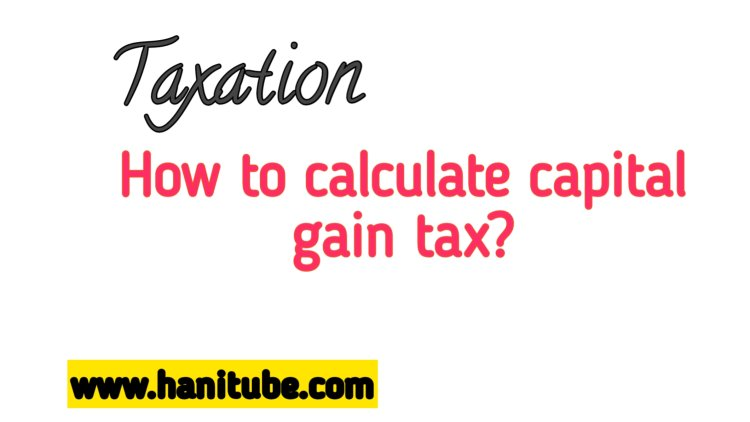# capital gains tax

## According to Ethiopian Tax Law capital gains tax is imposed on the income from the disposal of certain investment areas.       The income tax proclamation No. 979/2016 imposes a certain amount of tax on income from a disposal of certain investment areas. Article 59 (1) of the Proclamation, states that a person who derives a gain on the disposal of immovable property, a share, or bond (referred to as a “taxable asset’) is liable to pay an income tax.How to calculate the capital gain on immovable property like a building according to Ethiopia tax proclamation?

• According to Ethiopian Tax Law capital gains tax is imposed on the income from the disposal of certain investment areas.
• The income tax proclamation No. 979/2016 imposes a certain amount of tax on income from a disposal of certain investment areas. Article 59 (1) of the Proclamation, states that a person who derives a gain on the disposal of immovable property, a share, or bond (referred to as a “taxable asset’) is liable to pay an income tax.
• For the purpose of determining capital gains tax there are two types of taxable assets;

A) Class ‘A’ are immovable assets which includes a mining or petroleum right, or mining or petroleum information.

However, if the building is held and wholly used as a private residence for 2 years prior to the disposal of the asset, it is not considered an immovable asset and hence not subject to capital gains tax .

If the commercial building is considered an immovable asset, then it falls under Class 'A' taxable assets.

The capital gains tax rate for Class 'A' taxable assets is 15% .

B) Class ‘B’ taxable asset

• Means shares and bonds.
• The capital gains tax rate for Class 'A' taxable assets is 30%.

Calculate the capital gain on immovable property in Ethiopia can be done using the following steps, based on the Ethiopian tax proclamation:

Capital Gain = Net Sale Price - Adjusted Cost

Net Sale Price = Sales Price - Selling Expenses

Adjusted Cost =Acquisition Cost + (Asset Book Value) ∗ (Current Inflation Index / Base Year Inflation Index)

capital Tax= capital gain*Tax Rate

1) Determine the Acquisition(initial) Cost

This includes the

• purchase price,
• legal fees,
• registration costs,
• transfer taxes, and any other expenses directly related to the acquisition.

2) Determine Book value of the asset

Book value=Acquisition Cost -Accumulated Depreciation

• It is necessary to adjust the acquisition cost for inflation.
• The proclamation provides an inflation adjustment index, which you can use to calculate the adjusted cost.

·   Adjusted Cost =(Asset Book Value) x (Current Inflation Index / Base Year Inflation Index)

4) Consider Improvements: If any major improvements or additions were made to the property, their costs can be added to the adjusted cost. These costs should be direct expenditures related to improving the property's value.

Deduct any expenses incurred during the sales process, such as agent fees, legal fees, and advertising costs.

Net Sale Price = Sales Price - Selling Expenses

5) Calculate Sales Price: Determine the selling price of the property. This is the amount you received when you sold the property.

6) Deduct Expenses:

7) Calculate the Capital Gain: To calculate the capital gain, subtract the adjusted cost (including improvements) from the net sale price.

Capital Gain = Net Sale Price - Adjusted Cost

8) Apply the Tax Rate:

• The Ethiopian tax proclamation specifies a flat tax rate for capital gains on immovable property.
• Currently, it is set at 15%. Multiply the capital gain by the tax rate to get the tax liability.
• Tax Liability = Capital Gain x 0.15

Example

For example, suppose you bought a small building for ETB 5,000,000 and built a commercial building on it for ETB 60,000,000. You have been claiming depreciation on the building while declaring your taxes for the last 3 years. Later on, you sell the building for ETB 100,000,000 and incur 100,000 ETB in selling expenses.

• Let’s assume that your accumulated depreciation over these years is ETB 9,750,000 and
• Suppose you also made improvements to the building worth 200,000 ETB.
• The base year inflation index is 100, and the current inflation index is 150

Calculate

1) Capital Gain

2) Capital Gain Tax

Solution.

1) Determine the Acquisition Cost:

=5,000,000+60,000,000

=The acquisition cost is 65,000,000 ETB.

To adjust for inflation, we calculate the adjusted cost using the inflation indexes:

• Adjusted Cost =Asset Book Value x (Current Inflation Index / Base Year Inflation Index)
• Book Value =65,000,000-9,750,000= 55,250,000
• Adjusted Cost =55,250,000 x (150 / 100)

3) Consider Improvements: Add the cost of improvements to the adjusted cost:

• Adjusted Cost = 82,875,000. ETB + 200,000 ETB =83,075,000 ETB

4) Calculate Sales Price:

Net sale price=sales price- selling expenses

Net Sale Price = 100,000,000 ETB - 100,000 ETB

Net Sale Price = 99,900,000 ETB

5) Calculate the Capital Gain:

Capital Gain =net sale price- adjusted cost

Capital Gain = 99,900,000 ET - 83,075,000 ETB

Capital Gain= 16,825,000 ETB

6) Apply the Tax Rate

• The tax rate for capital gains on immovable property is 15%.
• Multiply the capital gain by the tax rate:
• Tax Liability = 16,825,000 ETB x 0.15 = 2,523,750 ETB